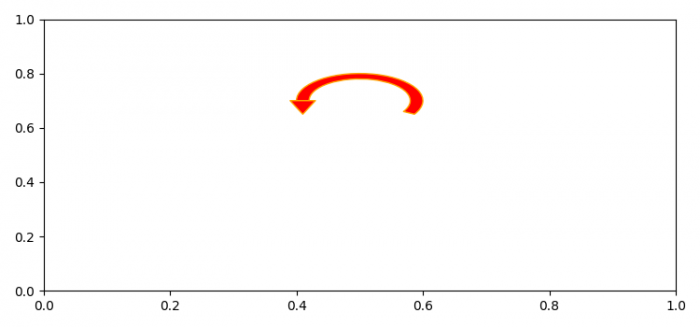# How to make an arrow that loops in Matplotlib?

To make an arrow that loops in Matplotlib, we can take the following steps −

• Set the figure size and adjust the padding between and around the subplots.
• To make an arrow loop in matplotlib, we can use make_loop() method.
• Make a wedge instance with center, radius, theta1, theta2 and width.
• To put the arrow top of the loop, use PathCollection.
• Add patch collection to the current axes.
• To display the figure, use show() method.

## Example

from matplotlib import pyplot as plt, patches, collections

plt.rcParams["figure.figsize"] = [7.50, 3.50]
plt.rcParams["figure.autolayout"] = True

rwidth = 0.02
ring = patches.Wedge(center, radius, theta1, theta2, width=rwidth)
offset = 0.02
xcent = center - radius + (rwidth / 2)
left = [xcent - offset, center]
right = [xcent + offset, center]
bottom = [(left + right) / 2., center - 0.05]
arrow = plt.Polygon([left, right, bottom, left])
p = collections.PatchCollection(
[ring, arrow],
edgecolor='orange',
facecolor='red'
)
plt.show()